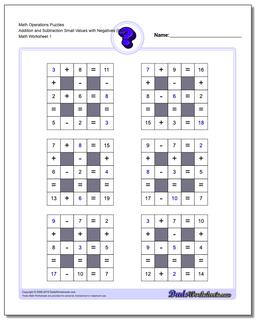# Math Worksheets: Number Grid Puzzles: Number Grid Puzzles: Math Operations Puzzles Addition and Subtraction Small Values with Negatives (Easy)## Math Operations Puzzles Addition and Subtraction Small Values with Negatives (Easy)

PropertyValue
DescriptionMath Operations Puzzles Addition and Subtraction Small Values with Negatives (Easy): Math puzzle worksheets that require students to fill in missing numbers with smaller numbers.
Resource TypeWorksheet